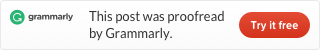# LeetCode problem 438 Find All Anagrams in a String (Java)

Given a string s and a non-empty string p, find all the start indices of p‘s anagrams in s.

Strings consists of lowercase English letters only and the length of both strings s and p will not be larger than 20,100.

The order of output does not matter.

Example 1:

Input:
s: “cbaebabacd”
p: “abc”
Output: [0, 6]
Explanation:
The substring with start index = 0 is “cba”, which is an anagram of “abc”.
The substring with start index = 6 is “bac”, which is an anagram of “abc”.

Example 2:

Input:
s: “abab”
p: “ab”
Output: [0, 1, 2]
Explanation:
The substring with start index = 0 is “ab”, which is an anagram of “ab”.
The substring with start index = 1 is “ba”, which is an anagram of “ab”.
The substring with start index = 2 is “ab”, which is an anagram of “ab”.

This problem is familiar with problem 76. And because the length of the anagram is equal with the origin string, this is a fix-width sliding window problem.

We can copy codes from problem 76 only change serval lines.

This is the code.

Running time is 6ms.